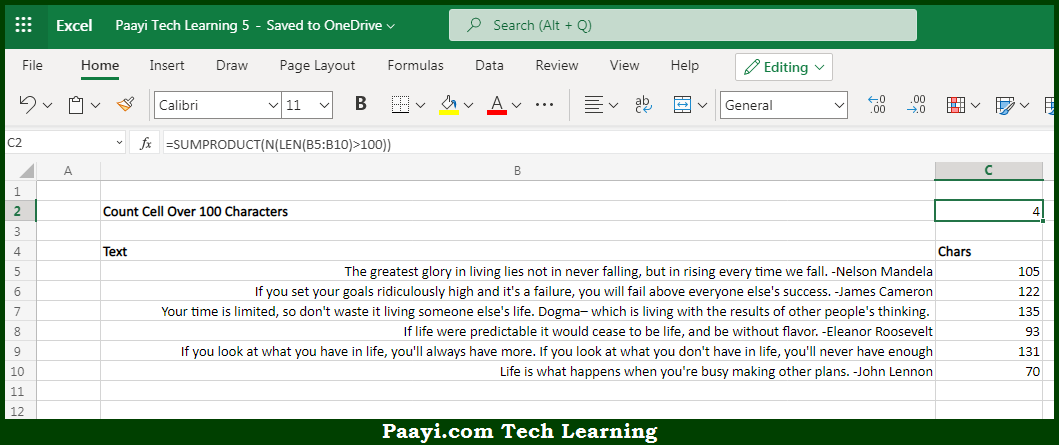# COUNT Cells Over 100 Characters in Microsoft Excel

Written by | 0 Comments | 554 Views

In this article, you will learn how to COUNT various things in Microsoft Excel using a single or combination of functions and its purpose in Microsoft Excel. You will also get to know how to count cells over 100 characters and see the generic formula.

Count Cells Over 100 Characters in Microsoft Excel

The main purpose of this formula is to count cells that contain more than a certain number of characters. Here we will learn how to count cells over 100 characters in Microsoft Excel.  That implies, with the help of a formula based on the  SUMPRODUCT, LEN, and N functions you can able to count cells that contain more than a certain number of characters. So, with the help of this formula, you can able to count cells not equal to any particular thing.

General Formula to COUNT Cells Over 100 Characters

=SUMPRODUCT(N(LEN(range)>100))

The Explanation for the COUNT Cells Over 100 CharactersSo we know that Microsoft Excel COUNTIF function you can able to count cells that contain more than a certain number of characters. Here we will learn how to count cells over 100 characters in Microsoft Excel. As we know that, the LEN function is used to run on the specified range, because we give LEN multiple values, it returns multiple results. It should be noted that every TRUE corresponds to a cell that contains more than 100  characters. Besides that, the N function converts these values to ones and zeros. So, with the help of the COUNT formula, you can able to count cells not equal to x or y.